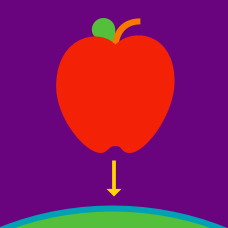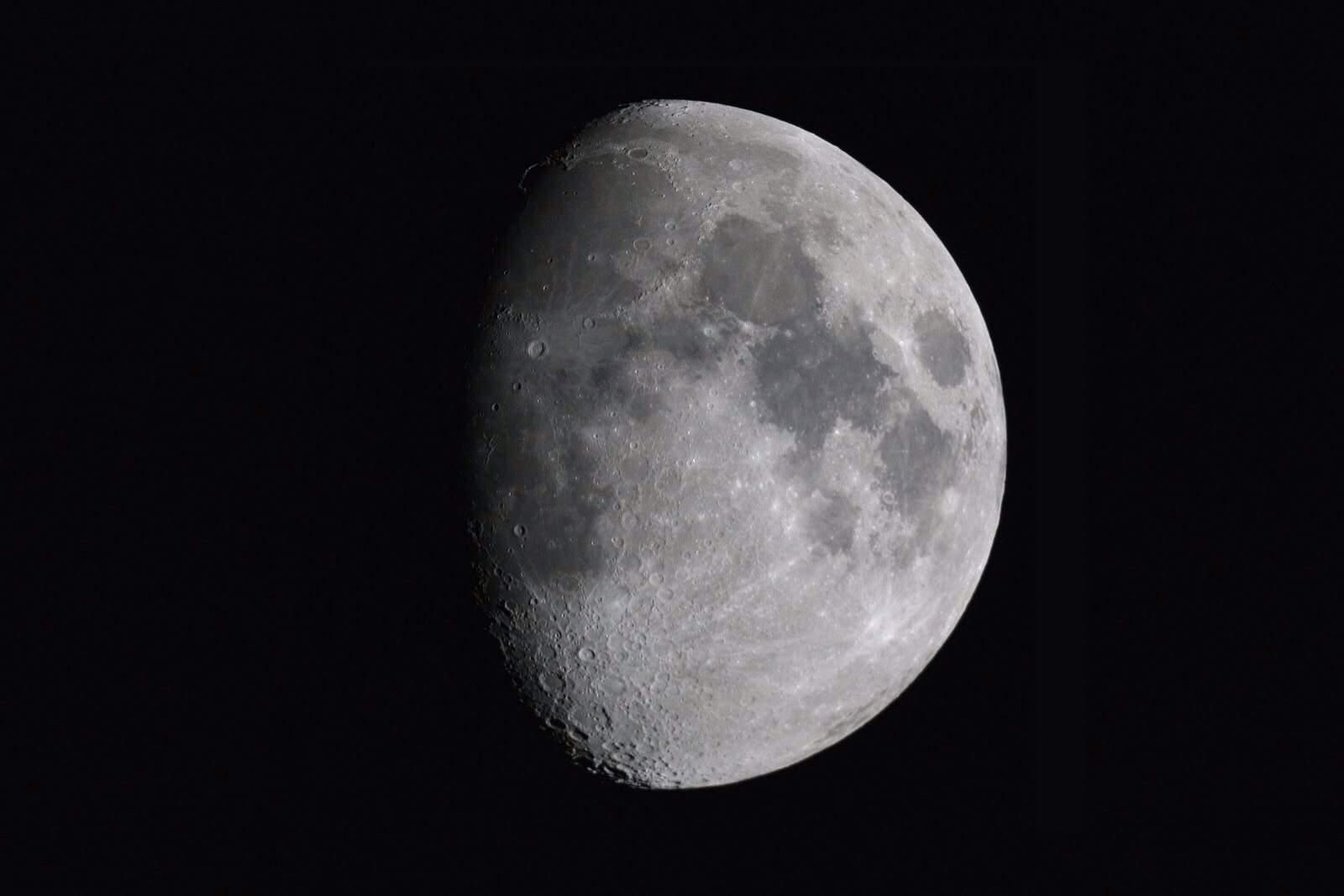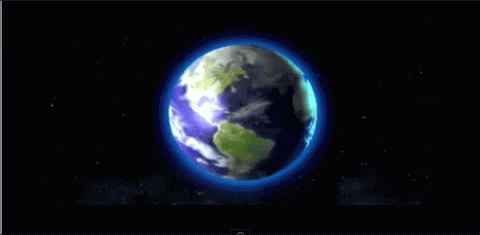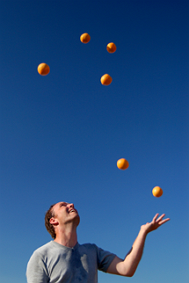Classical Mechanics

# Newton's Law of Gravity: Level 2-4 ChallengesAn object weighs $$30 \text{N}$$ on Earth. A second object weighs $$30 \text{N}$$ on the moon. Which has the greater mass?Sam the rock climber can climb his favorite route at the gym 20 times before he runs out of energy. Approximately how many times would he be able to climb the same route if it were built on Mars?


Details and Assumptions:

• The radius of Mars is 53% the radius of Earth.
• The mass of Mars is 11% the mass of Earth.
• When climbing on Mars, he has an air supply with the same composition as the Earth's air.

Consider a small black ball of radius $$R$$ and density $$\rho= 1~\frac{\mbox{g}}{\mbox{cm}^{3}}$$ located at a certain distance above the surface of the Sun. For what radius $$R$$ in micrometers is the gravitational attraction of the Sun counterbalanced by the radiation force?

Assume that the black ball absorbs all the incident light and that the total power radiated by the Sun is $$P=4\times 10^{26}~\mbox{W}$$.

Details and assumptions

The mass of the Sun is $$M_{s}=2\times 10^{30}~\mbox{kg}$$. The universal constant of gravitation is $$G=6.67\times 10^{-11}~\text{m}^{3}\text{kg}^{-1} \text{s}^{-2}$$ and the speed of light $$c=3\times 10^{8}~\mbox{m/s}$$.What is the minimum amount of energy required to irreversibly blow up our lovely planet into smithereens?

Details and assumptions

• Input your answer in Joules

• Model the Earth as sphere of uniform volume density composed of tiny neutral pieces of mass.

• The radius of the Earth is $$R_e=6371km$$.

• The mass of the Earth is $$M_e = 5.972\times 10^{24} kg$$

• Newton's gravitational constant is $$G=6.673\times10^{-11} m^3 kg^{-1} s^{-2}$$A ball is thrown up from the ground with an initial velocity of 10 meters per second. What is the maximum height (in meters) that it can reach?

Ignore air resistance and take the acceleration due to gravity as $$g=-10 \text{ m/s}^2$$.

×

Problem Loading...

Note Loading...

Set Loading...